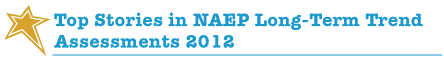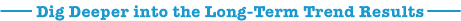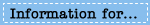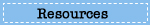The NAEP long-term trend assessments have monitored student progress in reading and mathematics for nationally representative samples of
9-, 13-, and 17-year-olds since the early 1970s. Results from the 2012 assessments show the following:

• Compared to the first assessment in 1971 for reading and in 1973 for mathematics, scores were higher in 2012 for 9- and 13-year-olds and not significantly different for 17-year-olds.
•

• In both reading and mathematics at all three ages, Black students made larger gains from the early 1970s than White students.
•

• Hispanic students made larger gains from the 1970s than White students in reading at all three ages and in mathematics at ages 13 and 17.
•

• Female students have consistently outscored male students in reading at all three ages, but the gender gap narrowed from 1971 to 2012 at age 9.
•

• At ages 9 and 13, the scores of male and female students were not significantly different in mathematics, but the gender gap in mathematics for 17-year-olds narrowed in comparison to 1973.Explore the following slides to take a closer look at the gains made by male and female students in reading and mathematics, and how performance gaps have narrowed for some age groups.

Slide 1

In 2012, male students made a larger gain in their average scores than their female counterparts compared to 1971. This was reflected in a narrowing of the gender gap from 13 points in 1971 to 5 points in 2012.

Changes in NAEP reading average scores for 9-year-olds, by gender, 1971 and 2012

The image shows a bar chart with 3 vertical bars. The ‘Y’ axis of the chart lists a range of scale scores from 0 to 500. The ‘X’ axis of the chart labels the bars as “all students,” “male” and “female,” respectively.  The top of each bar is labeled “2012” and the bottom of each is labeled “1971.”  The bars illustrate that the score change between 1971 and 2012 was 13 points for all students, 17 points for male students, and 10 points for female students. All the score changes were significantly different between the two assessment years.

Slide 2

In 2012, male students made a 9-point gain and female students made a 6-point gain compared to 1971; however, the score gap between male and female students in 2012 (8 points) was not significantly different from the score gap in 1971 (11 points).

Changes in NAEP reading average scores for 13-year-olds, by gender, 1971 and 2012

The image shows a bar chart with 3 vertical bars. The ‘Y’ axis of the chart lists a range of scale scores from 0 to 500. The ‘X’ axis of the chart labels the bars as “all students,” “male” and “female,” respectively.  The top of each bar is labeled “2012” and the bottom of each is labeled “1971.”  The bars illustrate that the score change between 1971 and 2012 was 8 points for all students, 9 points for male students, and 6 points for female students. All the score changes were significantly different between the two assessment years.

Slide 3

In 2012, male students made a 4-point gain compared to 1971, while scores for female students were about the same when comparing the two assessment years. The score gap between male and female students in 2012 (8 points) was not significantly different from the score gap in 1971 (12 points).

Changes in NAEP reading average scores for 17-year-olds, by gender, 1971 and 2012

The image shows a bar chart with 3 vertical bars. The ‘Y’ axis of the chart lists a range of scale scores from 0 to 500. The ‘X’ axis of the chart labels the bars as “all students,” “male” and “female,” respectively.  The top of each bar is labeled “2012” and the bottom of each is labeled “1971.”  The bars illustrate that the score change between 1971 and 2012 was 2 points for all students, 4 points for male students, and rounds to zero points for female students. The score change for male students was significantly different between the two assessment years.

Slide 4

Mathematics: Age 9

In 2012, male students made a 26-point gain and female students made a 24-point gain compared to 1973. There were no significant differences between the average mathematics scores for male and female students in 1973 or 2012.

Changes in NAEP mathematics average scores for 9-year-olds, by gender, 1973 and 2012

The image shows a bar chart with 3 vertical bars. The ‘Y’ axis of the chart lists a range of scale scores from 0 to 500. The ‘X’ axis of the chart labels the bars as “all students,” “male” and “female,” respectively.  The top of each bar is labeled “2012” and the bottom of each is labeled “1973.”  The bars illustrate that the score change between 1973 and 2012 was 25 points for all students, 26 points for male students, and 24 points for female students. All the score changes were significantly different between the two assessment years.

Slide 5

Mathematics: Age 13

In 2012, male students made a 21-point gain and female students made a 17-point gain compared to 1973. There were no significant differences between the average mathematics scores for male and female students in 1973 or 2012.

Changes in NAEP mathematics average scores for 13-year-olds, by gender, 1973 and 2012

The image shows a bar chart with 3 vertical bars. The ‘Y’ axis of the chart lists a range of scale scores from 0 to 500. The ‘X’ axis of the chart labels the bars as “all students,” “male” and “female,” respectively.  The top of each bar is labeled “2012” and the bottom of each is labeled “1973.”  The bars illustrate that the score change between 1973 and 2012 was 19 points for all students, 21 points for male students, and 17 points for female students. All the score changes were significantly different between the two assessment years.

Slide 6

Mathematics: Age 17

In 2012, female students made a 3-point gain compared to 1973, while scores for male students were not significantly different when comparing the two assessment years. The male-female score gap narrowed from 8 points in 1973 to 4 points in 2012.

Changes in NAEP mathematics average scores for 17-year-olds, by gender, 1973 and 2012

The image shows a bar chart with 3 vertical bars. The ‘Y’ axis of the chart lists a range of scale scores from 0 to 500. The ‘X’ axis of the chart labels the bars as “all students,” “male” and “female,” respectively.  The top of each bar is labeled “2012” and the bottom of each is labeled “1973.”  The bars illustrate that the score change between 1973 and 2012 was 2 points for all students, -1 points for male students, and 3 points for female students. The score change for female students was significantly different between the two assessment years.

# Rounds to zero.
* Score change was significantly different (p < .05) between the two assessment years.
SOURCE: U.S. Department of Education, Institute of Education Sciences, National Center for Education Statistics, National Assessment of Educational Progress (NAEP), 1971, 1973, and 2012 Long-Term Trend Reading and Mathematics Assessments.

VIEW NON-FLASH VERSION

Explore the 2012 Long-Term Trend Report Card••